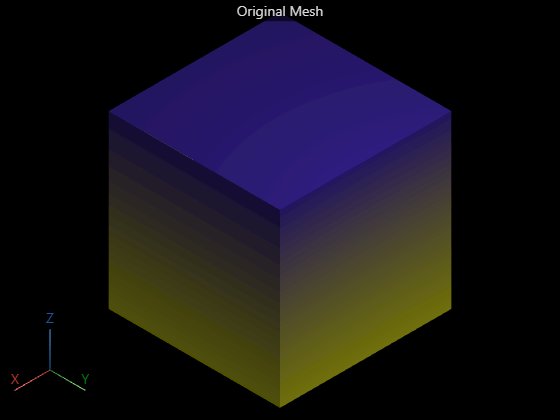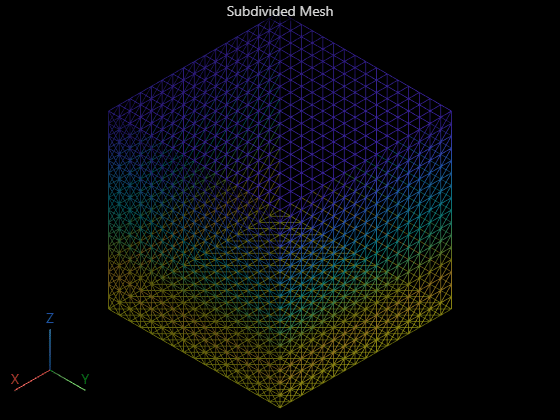# simplify

Simplify surface mesh

Since R2022b

## Syntax

``simplify(mesh)``
``simplify(mesh,Name=Value)``

## Description

example

````simplify(mesh)` simplifies the surface mesh `mesh` by using quadric decimation.```

example

````simplify(mesh,Name=Value)` specifies options using one or more name-value arguments. For example, `SimplificationMethod="vertex-clustering"` simplifies the surface mesh by using the vertex-clustering method.```

## Examples

collapse all

Define mesh vertices for a surface mesh.

```vertices = [1 -1 1; 1 1 1; -1 1 1; -1 -1 1; ... 1 -1 -1; 1 1 -1; -1 1 -1; -1 -1 -1];```

Define the mesh faces using the vertices.

```faces = [6 2 1; 1 5 6; 8 4 3; 3 7 8; 6 7 3; 3 2 6; ... 5 1 4; 4 8 5; 4 1 2; 2 3 4; 7 6 5; 5 8 7];```

Create and display the surface mesh.

```mesh = surfaceMesh(vertices,faces); surfaceMeshShow(mesh,Title="Original Mesh")```Subdivide the mesh using the midpoint-split method, and display the subdivided mesh.

```numIterations = 4; subdivide(mesh,"midpoint-split",numIterations) surfaceMeshShow(mesh,Title="Subdivided Mesh",WireFrame=true)```Simplify the surface mesh by using the quadric-decimation method.

``` simplify(mesh,SimplificationMethod="quadric-decimation", ... TargetNumFaces=30)```

Remove any unreferenced vertices, and display the simplified mesh.

```removeDefects(mesh,"unreferenced-vertices") surfaceMeshShow(mesh,Title="Simplified Mesh",WireFrame=true)```Read a surface mesh from an STL file.

```fileName = fullfile(toolboxdir("lidar" ),"lidardata", ... "surfaceMesh","mobius.stl"); mesh = readSurfaceMesh(fileName);```

Display the surface mesh.

`surfaceMeshShow(mesh,Title="Original Mesh",WireFrame=true)`Simplify the surface mesh by using the vertex-clustering method.

```simplify(mesh,SimplificationMethod="vertex-clustering", ... VoxelSize=0.15,MergeMethod="Quadric")```

Remove any unreferenced vertices, and display the simplified mesh.

```removeDefects(mesh,"unreferenced-vertices") surfaceMeshShow(mesh,Title="Simplified Mesh",WireFrame=true)```## Input Arguments

collapse all

Surface mesh, specified as a `surfaceMesh` object.

### Name-Value Arguments

Specify optional pairs of arguments as `Name1=Value1,...,NameN=ValueN`, where `Name` is the argument name and `Value` is the corresponding value. Name-value arguments must appear after other arguments, but the order of the pairs does not matter.

Example: `simplify(mesh,SimplificationMethod="vertex-clustering")` simplifies the surface mesh by using the vertex-clustering method.

Surface mesh simplification method, specified as `"quadric-decimation"` or `"vertex-clustering"`. For more information on these simplification methods, see the Simplification Methods section.

Data Types: `char` | `string`

Number of triangular faces in the simplified mesh, specified as a positive integer. By default, the function computes this values as `0.2` times the number of faces in the input mesh.

Note

This argument is applicable only when you specify `SimplificationMethod` as `"quadric-decimation"`.

Data Types: `single` | `double` | `int32` | `uint32`

Maximum allowed error when a vertex is merged, specified as a positive scalar.

Note

This argument is applicable only when you specify `SimplificationMethod` as `"quadric-decimation"`.

Data Types: `single` | `double`

Weight for the edge vertices, specified as a positive scalar. The function uses this value to preserve the mesh boundaries.

Note

This argument is applicable only when you specify `SimplificationMethod` as `"quadric-decimation"`.

Data Types: `single` | `double`

Voxel size for vertex clustering, specified as a positive scalar. The function pools all the vertices within the specified voxel size to form clusters.

Note

This argument is applicable only when you specify `SimplificationMethod` as `"vertex-clustering"`.

Data Types: `single` | `double`

Vertex merging method, specified as `"Average"` or `"Quadric"`. When the value is specified as `"Average"`, the function computes an average value of all vertices in a voxel. When the value is specified as `"Quadric"`, the function minimizes the distance between the adjacent planes to merge the vertices.

Note

This argument is applicable only when you specify `SimplificationMethod` as `"voxel-clustering"`.

Data Types: `char` | `string`

## Limitations

The function discards the `FaceColors` property of the input `surfaceMesh` object. The simplified mesh does not have any face colors.

## Algorithms

Quadric decimation is a method that uses iterative contractions of vertex pairs to simplify a mesh, and maintains error approximation for all vertices using quadric matrices. The method consists of these steps.

1. Compute quadric matrices for all vertices, and select vertex pairs to merge.

2. Compute the contraction target vertex for each pair.

3. Iteratively minimize the error of the target vertices to construct the simplified mesh.

Vertex clustering is a method that uses bounding boxes to divide the mesh into voxels. The function clusters the vertices in each voxel into a single vertex and then updates the mesh faces accordingly.

## Version History

Introduced in R2022b

expand all